Power of a Product

When a product is inside the parentheses and a power is on the outside of the parentheses, then the outside power multiplies the exponent of each element inside.

Power of a Product Rule: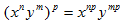*Note: This rule applies to a product only. If there is a sum or difference inside, refer to the steps of multiplying binomials.

Example 1 Simplify the following expression: (x2y)4

Step 1. Rewrite the expression with each element inside the parentheses to the power on the outside.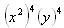Step 2. Use the Power of a Power Rule.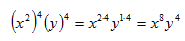Example 2 Simplify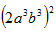.

Use the Power of a Product Rule to simplify the expression.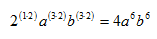Listen